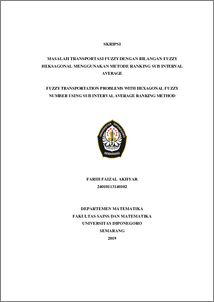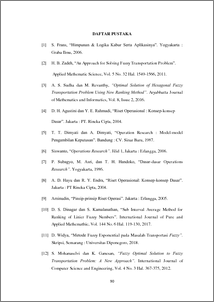# FUZZY TRANSPORTATION PROBLEMS WITH HEXAGONAL FUZZY NUMBER USING SUB INTERVAL AVERAGE RANKING METHOD

Akhyar, Farih Faizal (2019) FUZZY TRANSPORTATION PROBLEMS WITH HEXAGONAL FUZZY NUMBER USING SUB INTERVAL AVERAGE RANKING METHOD. Undergraduate thesis, UNDIP.Preview
PDF
154KbPDF
Restricted to Repository staff only

1035KbPreview
PDF
166Kb

## Abstract

The process of distributing goods from one place to another or what we know as transportation problems, always has various obstacles that ultimately affect the amount of profits obtained by business actors. Fuzzy transportation is a transportation problem where the cost of transportation, the amount of inventory and the amount of demand is fuzzy. One of the fuzzy transportation problems that often arises is the hexagonal fuzzy transportation problem where the fuzzy transportation problem has parameters in the form of blurring of six numbers. The hexagonal fuzzy transportation problem can be solved by changing all the parameters of the hexagonal fuzzy number into firm numbers using the Sub Interval Average ranking method. After the firm numbers are obtained, the problem is solved by the Least-Cost method to obtain a feasible solution, followed by the Modified Distribution method to obtain the optimal solution. Based on numerical simulations of fuzzy transportation problems with hexagonal fuzzy numbers, the Sub Interval Average ranking method is very well used as a method of affirming hexagonal fuzzy numbers, and the Least-Cost method, followed by the Modified Distribution method producing an optimal solution with a simpler process than other methods. Keyword : Fuzzy transportation, hexagonal fuzzy, Sub Interval Average method, Least-Cost method, Modified Distribution method.

Item Type: Thesis (Undergraduate) Q Science > QA Mathematics Faculty of Science and Mathematics > Department of Mathematics 84248 INVALID USER 13 Jun 2022 08:53 13 Jun 2022 08:53

Repository Staff Only: item control page